# HYPERBOLIC FUNCTIONS Solutions Inter Maths 1B

Inter first year Maths 1A textbook chapter 9 Hyperbolic Functions exercise 9(a) solutions are given.

You study textbook lesson Hyperbolic functons very well.

Observe the example problems and solutions given in the textbook.

Observe the given below solutions and try them in your own method.

You can also see the solutions

SSC Maths text book Solutions class 10

Inter maths 1A textbook solutions

Inter Maths 1B textbook solutions

Inter Maths 1IA text book solutions

Inter Maths IIB text book solutions

M

## TRIGONOMETRY HYPERBOLIC FUNCTIONS JUNIOR INTER

### Hyperbolic functions solutions

Exercise 9(a)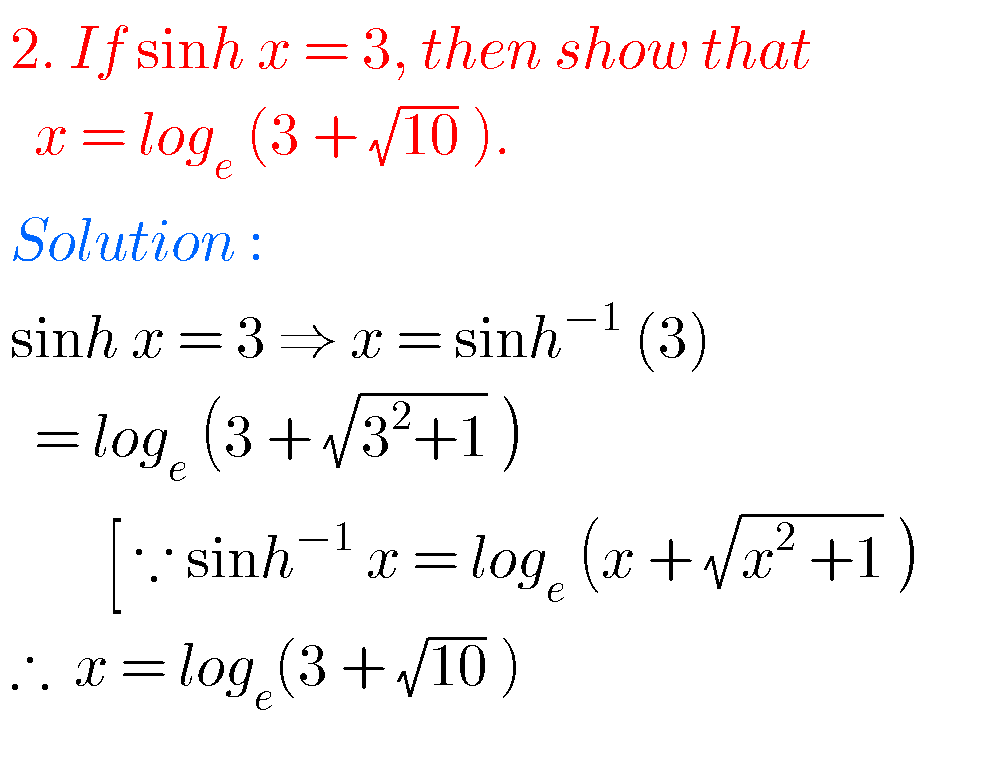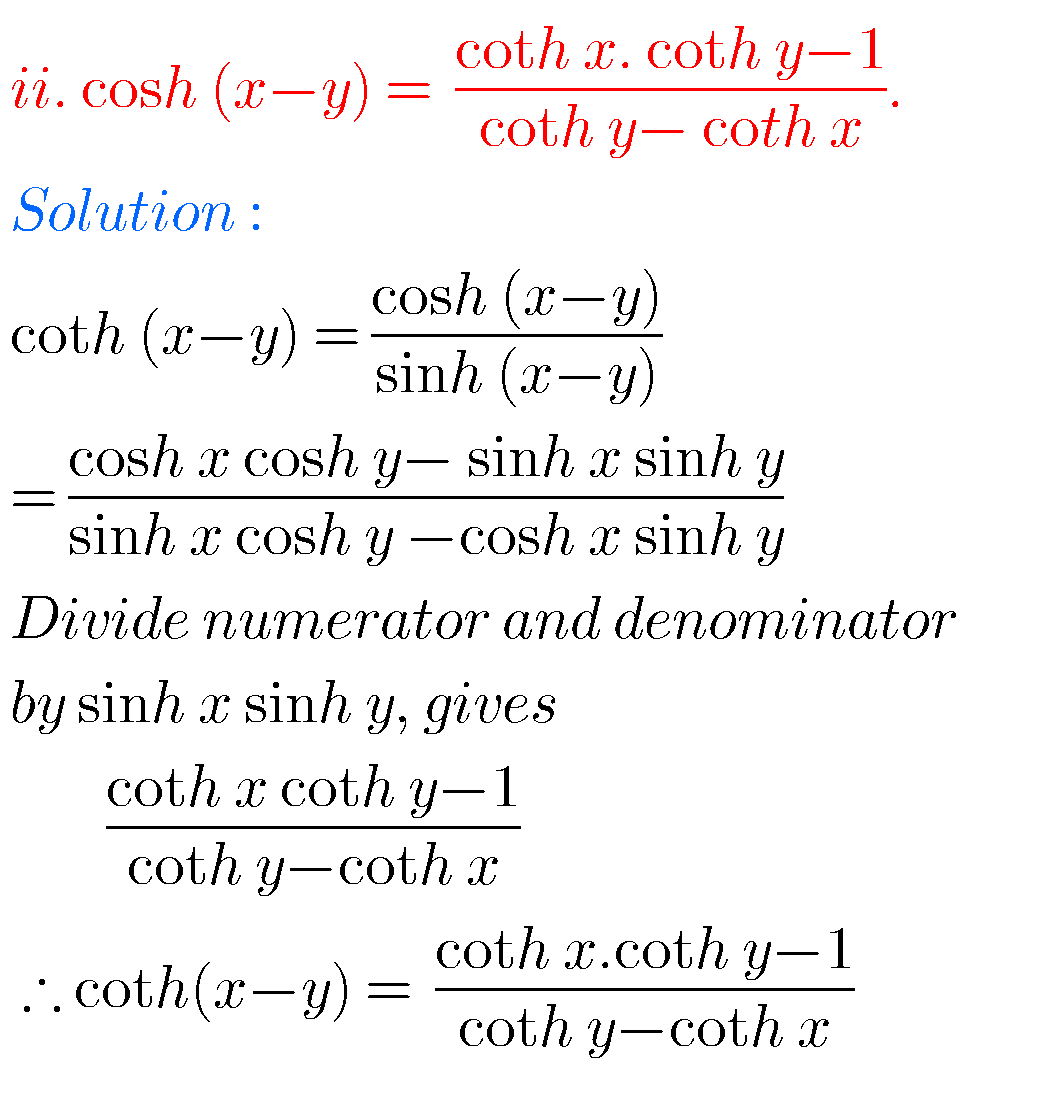# INTERMEDIATE FIRST YEAR 1 A PROBLEMS WITH SOLUTIONS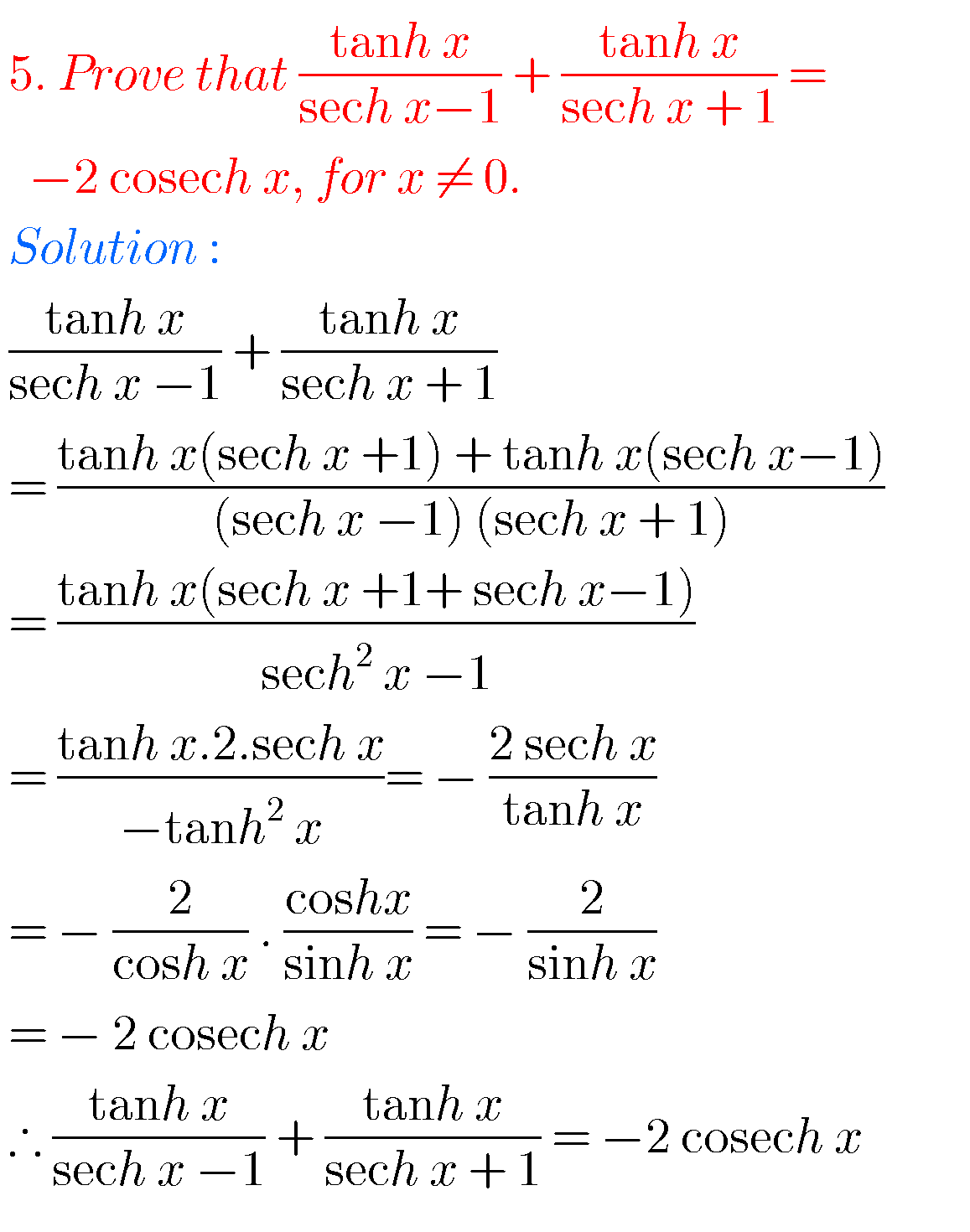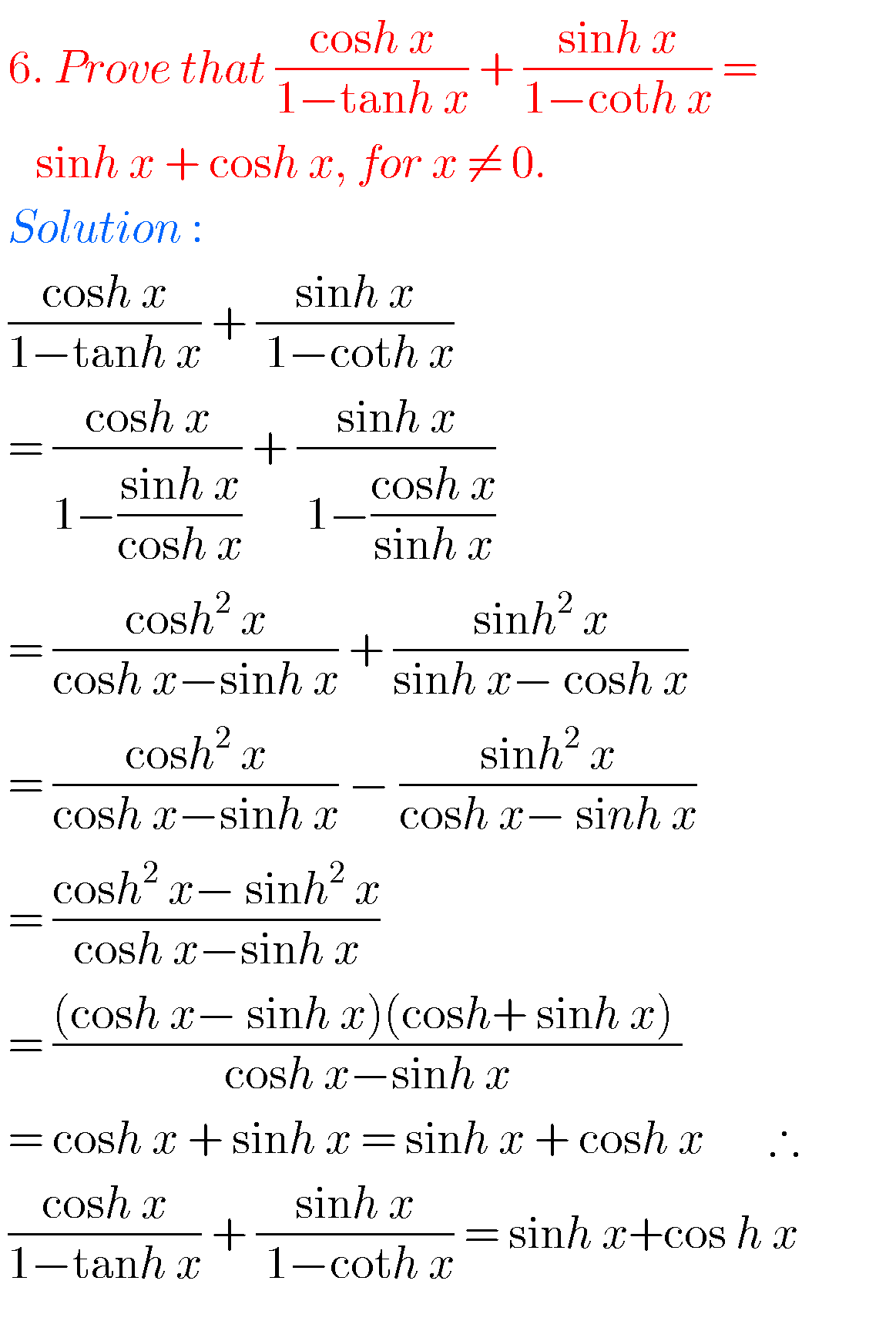## TRIGONOMETRY HYPERBOLIC FUNCTIONS JUNIOR INTER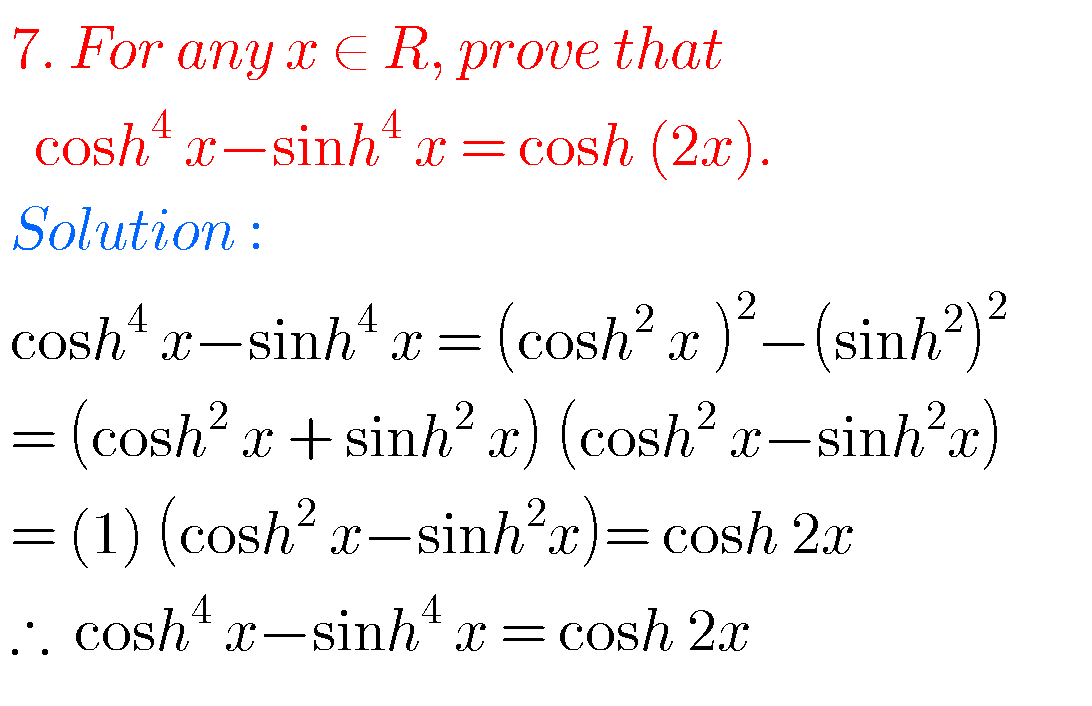Note : Observe the solutions of hyperbolic functions and try them in your own methods.

Some more solutions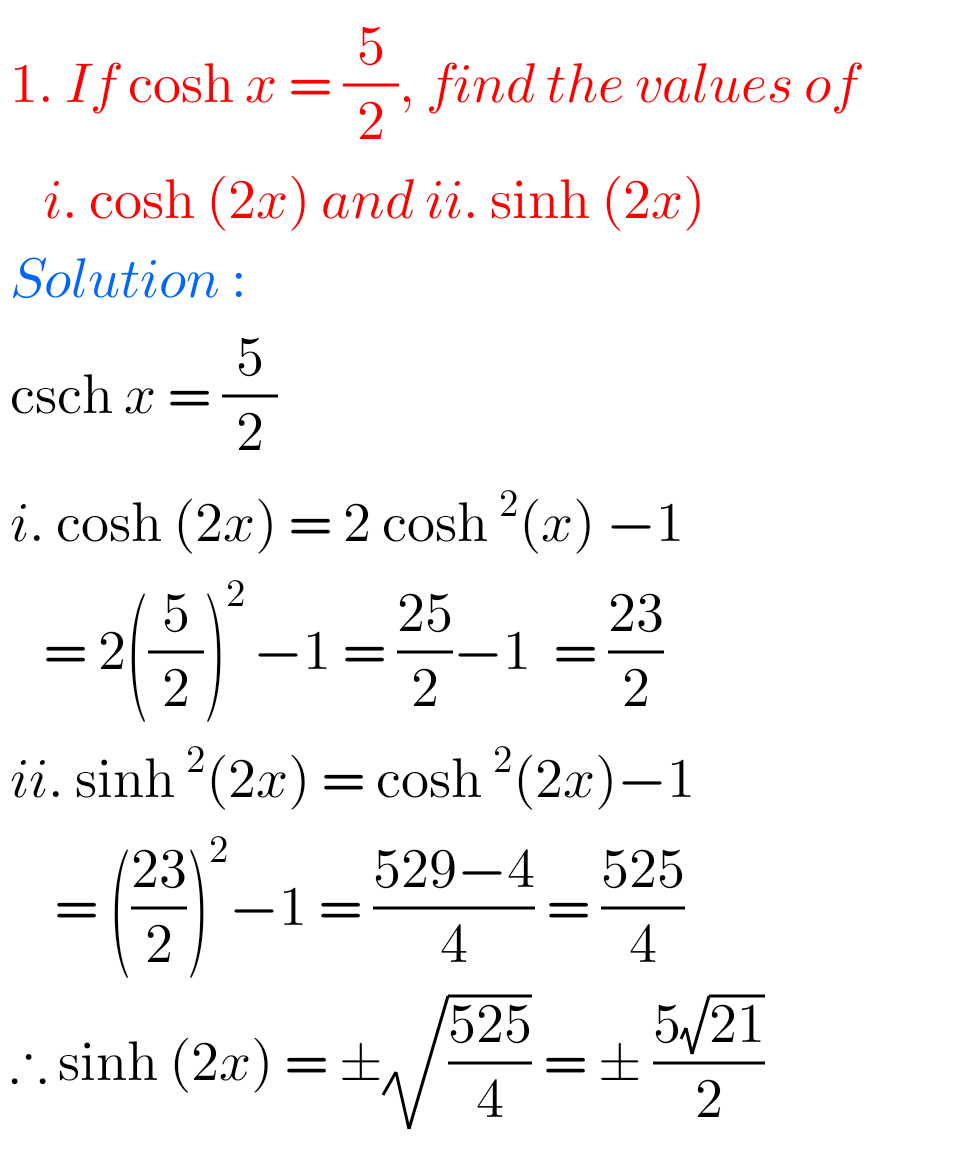Inter maths trigonometry solutions

Maths solutions class 10